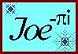Purlin or Square Fascia intersects Main Roof
Compound Angle Formulas in terms of Roof Pitch Angles
Main Ridge and Dormer Ridge intercept in Plan View = 90°

Purlin Face perpendicular to Dormer Roof
 Saw Blade Bevel = arcsin (cos Main Roof Pitch Angle × sin Dormer Pitch Angle) Saw Blade Miter = arctan (cos Dormer Pitch Angle ÷ tan Main Roof Pitch Angle) Angle on the Stick = arctan (tan Main Roof Pitch Angle ÷ cos Dormer Pitch Angle)
Purlin Face set in Dormer Roof
 Saw Blade Bevel = arcsin (cos Main Pitch Angle × cos Dormer Pitch Angle) Saw Blade Miter = arctan (sin Dormer Pitch Angle ÷ tan Main Pitch Angle) Angle on the Stick = arctan (tan Main Pitch Angle ÷ sin Dormer Pitch Angle)
Footprint Angle or Mortise Angle on the Main Roof Slope
 arctan [ tan Main Pitch Angle cos Main Pitch Angle × sin Dormer Pitch Angle × cos Dormer Pitch Angle ]